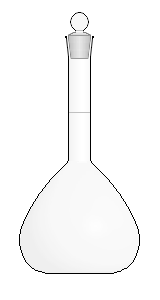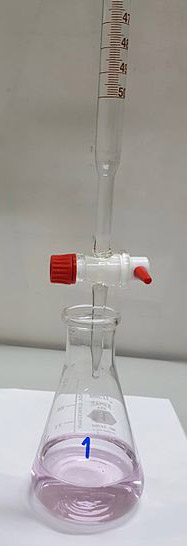Chemistry µGCSE:

Solution Moles

10 quick questions - for GCSE and iGCSE

10 minutes maximum!

Grab a calculator and Periodic Table - time for some maths!

1. Which of these is the correct formula for the number of moles in solution?
• A.   number of moles = concentration x volume in cm3
• B.   number of moles = concentration x volume in dm3
• C.   number of moles = concentration ÷ volume in cm3
• D.   number of moles = concentration ÷ volume in dm3
2. The amount, in moles, of KOH in 25.0cm3 of 0.150 mol/dm3 potassium hydroxide solution is …
• A.   3.75 mol
• B.   0.00375 mol
• C.   6.00 mol
• D.   0.00600 mol
3. The volume of 0.500 mol/dm3 sulfuric acid which contains 0.0450 moles of sulfuric acid is …
• A.   0.0225 cm3
• B.   0.0900 cm3
• C.   22.5 cm3
• D.   90.0 cm3

Q4+5.

A student made a standard solution of sodium hydroxide by dissolving 10.0g of sodium hydroxide, NaOH, in distilled water to make 250cm3 of solution.

[Mr: NaOH = 40]4. Calculate the amount, in moles, of sodium hydroxide used.
• A.   0.250
• B.   4.00
• C.   400
• D.   2.50
5. Calculate the concentration, in mol/dm3, of the sodium hydroxide solution produced.
• A.   0.00100 mol/dm3
• B.   0.0160 mol/dm3
• C.   1.00 mol/dm3
• D.   16.0 mol/dm3
6. Hydrobromic acid can be neutralized using potassium hydroxide solution.

The equation for the reaction is:

HBr(aq) + KOH(aq)  KBr(aq)  + H2O

Calculate the volume of 0.20 mol/dm3 potassium hydroxide solution needed to exactly neutralize 0.0020 moles hydrobromic acid.
• A.   0.010 cm3
• B.   1.0 cm3
• C.   0.010 dm3
• D.   10 dm3

Q7-10.
A chemist carries out a titration to find the concentration of a solution of sodium hydroxide.

She titrates 25.0cm3 of sodium hydroxide solution with a solution of sulfuric acid using phenolphthalein as an indicator.

14.6cm3 of 0.200mol/dm3 sulfuric acid was required.

The equation for the reaction is:

 2NaOH(aq) + H2SO4(aq) → Na2SO4(aq) + 2H2O(l)7. The most suitable pieces of apparatus for measuring the volumes of sodium hydroxide and sulfuric acid are:

 Sodium hydroxide volume Sulfuric acid volume A burette pipette B pipette burette C Measuring cylinder burette D Measuring cylinder Measuring cylinder
8. The amount, in moles, of sulfuric acid used in this titration is ….
• A.   0.00500
• B.   5.00
• C.   0.00292
• D.   2.92
9. The amount, in moles, of sodium hydroxide used to neutralize the sulfuric acid is …
• A.   0.00292
• B.   0.00584
• C.   0.00146
• D.   5.84
10. The concentration, in mol/dm3, of the sodium hydroxide solution used to neutralize this sulfuric acid is ….
• A.   0.234 mol/dm3
• B.   0.117 mol/dm3
• C.   0.0584 mol/dm3
• D.   0.400 mol/dm3